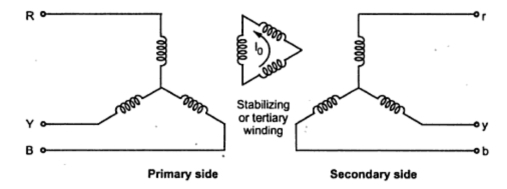### Three winding Transformers

1.1 Introduction
In addition to primary and secondary windings, the transformers may be constructed with the third winding. This winding is called tertiary winding. The normal two winding transformer can be converted into three winding transformer with an additional secondary winding having number of turns as per the requirements.

1.2 Why to use tertiary winding ?
There are many reasons for which three winding transformers is employed. Some of the reasons are listed below.
1. If a two winding transformer has to supply an additional load which has to be insulated from the secondary windings for some reasons then three winding transformer may used with additional load carried by tertiary winding.
2. The phase compensating devices can be supplied with three winding transformer which are not operating at either primary or secondary voltage but at some different voltage.
3. The tertiary winding can be used as a voltage coil in a testing transformer.
4. Three supply systems operating at different voltages can be interconnected using three winding transformer.
5. The three winding transformer can be used to load large split winding generators.
6. The substation requirements can be met using three winding transformer which requires a voltage different from that of primary and secondary windings.
7. The tertiary winding connected in delta reduces the impedance offered to the zero sequence currents so a larger earth fault current flows for proper operation of protective equipment. For unbalanced load it limits the imbalance in voltage. It permits the flow of third harmonic current to reduce third harmonic voltage.
The third winding known as tertiary winding is generally connected in delta. Thus when any fault or short circuit occurs on the primary or secondary sides, there will be large unbalance of phase voltage which is compressed by large tertiary winding circulating current. The reactance of the tertiary winding must be such as to limit the circulating current to that which can be carried by copper in order to avoid overheating of tertiary winding under fault conditions.
1.3 Stabilizing Due to Tertiary Winding
For unbalanced single phase load, the star-star connection offers high reactance to flow of current. Any unbalanced load current has three components viz positive, negative and zero sequence components. The zero sequence component on the secondary side can not be balanced by primary currents as zero sequence currents can not flow in the isolated neutral of star connected primary. On the secondary side the zero sequence current sets up magnetic flux in the core. The iron path is available for this flux and the impedance offered to the zero sequence currents is very high. But the delta connected tertiary winding permits circulation of zero sequence currents in it. So impedance offered to the flow of zero sequence currents is lowered. For this purpose the tertiary winding is called stabilizing winding. This is shown in the Fig. 1.Fig. 1

1.4 Equivalent Circuit of 3 Winding Transformer
While drawing the equivalent circuit of a three winding transformer, each of the winding is represented by its equivalent resistance and reactance. The exciting current is neglected this is shown in the Fig. 2.Fig. 2 Single phase equivalent circuit of three winding transformer

All the parameters values are reduced to a common rating base and respective voltage bases. The primary, secondary and tertiary windings are respectively indicated by subscripts 1, 2 and 3. The circuit is simplified by neglecting the exciting current.
The division of load between the secondary and tertiary winding is arbitrary. External circuits are respectively connected between terminals 1, 2, 3 and the common terminal.
As exciting current is neglected,
Ī1 + Ī2+ Ī3 = 0
The determination of impedance in the above equivalent circuit is carried put from three simple short circuit tests.

All the above impedances are referred to a common base from the equivalent circuit,

Subtracting equations (2) and (3)

Subtracting equations (4) and (1)

Similarly,

The open circuit test can be performed on any one of the three windings. This helps in obtaining the core loss, magnetizing impedance and turns ratio.
The advantages of a three winding transformer are as given below
ii) It can act as a source of voltage at substation to meet the internal load demand of substation which is at different voltage that either of primary or secondary voltage level.
iii) The reactive power injection into the system is possible for voltage control by connecting synchronous condensers or static capacitors to the tertiary winding.
iv) A delta connected tertiary winding offers less impedance to the flow of zero sequence currents. The allows larger earth fault current to flow through protective device facilitating its proper operation.
v) It reduces voltage unbalance under unbalanced loading conditions and permits third harmonic current to flow which reduces third harmonic voltages.
vi) Three transmission lines at different voltage levels can be interconnected by using three winding transformer.
vii) The third winding of a three winding transformer, usually called tertiary winding can be used to serve purpose of measuring voltage of HV testing transformer.
The disadvantage of a three winding transformer is its construction is little complicated as compared to normal two winding transformer. A separate third winding is required to be placed which requires more copper and hence cost of three winding transformer is obviously more. The core of the transformer has to carry three windings instead of two as in case of normal two winding transformer.

Solved Examples on 3Winding Transformer

Related Articles

1.